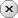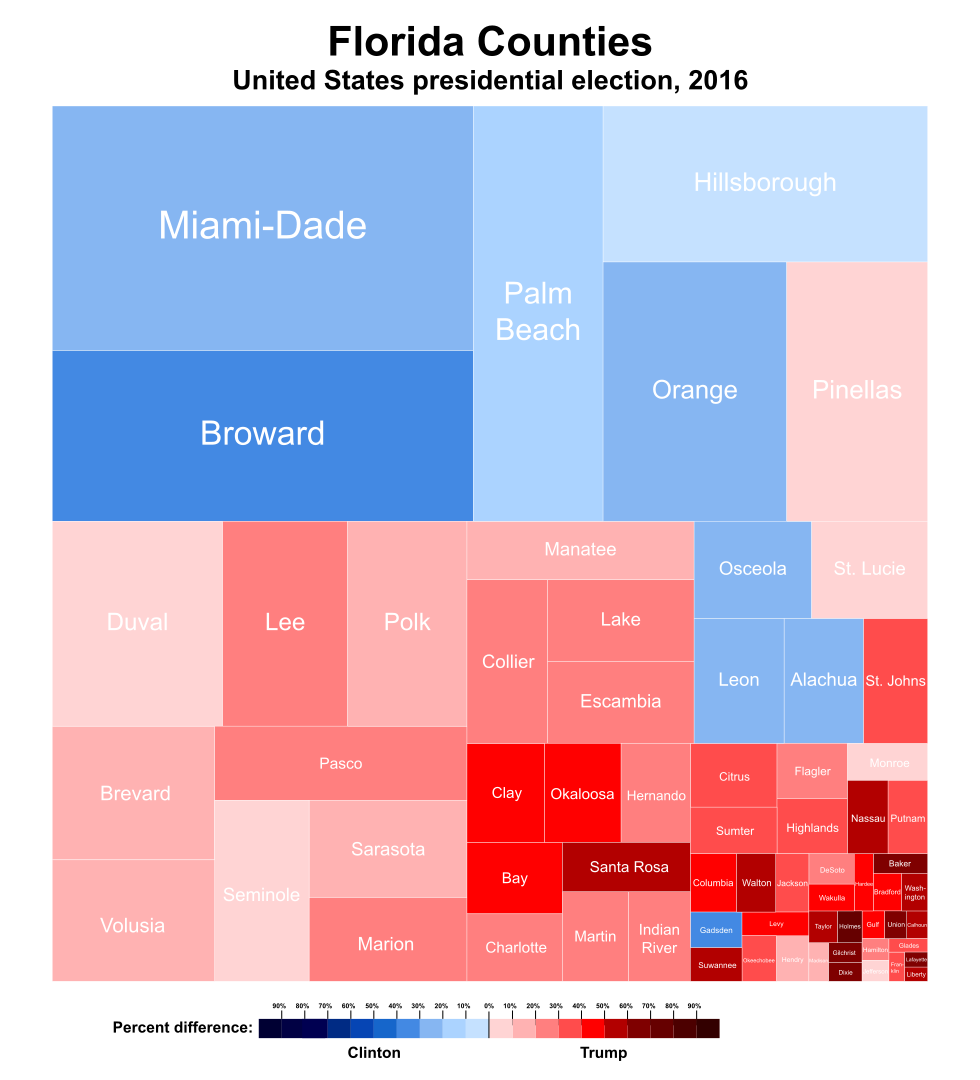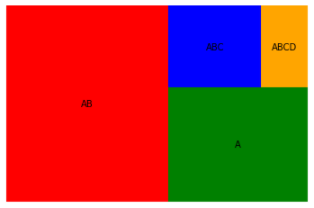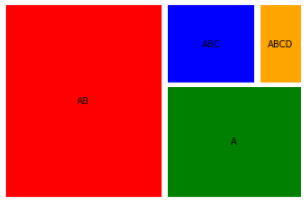### 用户注册### 用户登录### 发表随想Python自学    -  云代码空间

—— python自学——怎么学习python,自学python要多久？python+人工智能+人工智能+人工智能+大数据分析

# 一行 Python 代码轻松构建树状热力图（一种可视化技术：treemap）

2022-04-17|589阅||

## 介绍Python 中的，可以使用 Squarify 直接构建树状图。且只需要一行代码squarify.plot(data)即可轻松构建。## 1. 安装必要的库

`!pip install squarify`

## 2. 导入必要的库

`import matplotlib.pyplot as plt import squarify`

## 3. 创建随机数据

`随机生成一个值列表，这些值将作为数据传递到我们的绘图中。`
`data = [500, 250, 60, 120] `

## 4. 绘制树状图

```plt.axis('off')  隐藏 树状图 的轴。
squarify.plot(data)
plt.axis('off')
plt.show()```

## 5. 把它们放在一起

```import matplotlib.pyplot as plt import squarify
data = [500, 250, 60, 120]
squarify.plot(data)
plt.axis('off')
plt.show()```## 1. 指定树状图的颜色

```import matplotlib.pyplot as plt import squarify
sizes = [500, 250, 120, 60]
color = ['red', 'green', 'blue', 'orange']
squarify.plot(sizes,color=color)
plt.axis('off')
plt.show()```## 2.给树状图添加标签

```import matplotlib.pyplot as plt import squarify
labels = ['A', 'AB', 'ABC', 'ABCD']
sizes = [500, 250, 120, 60]
color = ['red', 'green', 'blue', 'orange']
squarify.plot(sizes,color=color, label = labels)
plt.axis('off')
plt.show()``````import matplotlib.pyplot as plt import squarify
labels = ['AB', 'A', 'ABC', 'ABCD']
sizes = [500, 250, 120, 60]
color = ['red', 'green', 'blue', 'orange']
squarify.plot(sizes,color=color, label = labels, pad = True)
plt.axis('off')
plt.show()```## 写在最后

plotly库。在今天的次条推文中介绍了其应用案例，感兴趣的小伙伴可以看看。

```import plotly_express as px import plotly.graph_objects as go
px.treemap(names = name, parents = parent)
go.Figure(go.Treemap(labels = name, parents = parent,))```

发表评论

## 个人资料

• 昵称： Python自学
• 等级： 高级设计师
• 积分： 5139
• 代码： 428 个
• 文章： 26 篇
• 随想： 4 条
• 访问： 103 次
• 关注